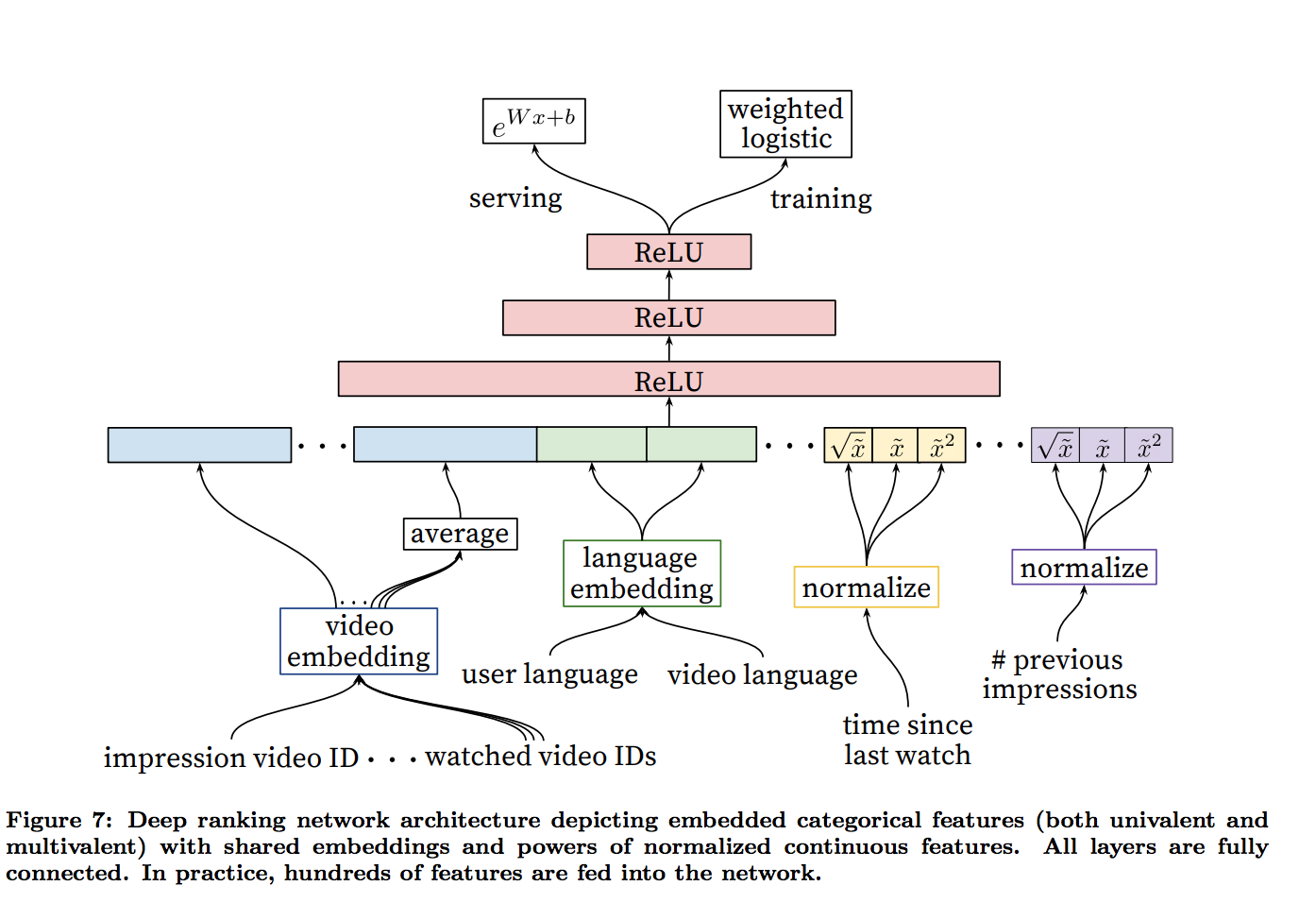To Top

Deep neural networks for youtube recommendations

## 候选生成网络（Candidate Generation Network）

### 将推荐看成分类问题

$P(w_t=i|U,C)=\frac{e^{v_iu}}{\sum _{j\in V}e^{v_ju}}$

item-embedding也可以参考https://zhuanlan.zhihu.com/p/24339183?refer=deeplearning-surfing里说的Item2vec: Neural Item Embedding for Collaborative Filtering的想法，把item视为word，用户的行为序列视为一个集合，item间的共现为正样本，并按照item的频率分布进行负样本采样，相似度的计算还只是利用到了item共现信息，缺点是：忽略了user行为序列信息; 没有建模用户对不同item的喜欢程度高低。

### 4.2 Modeling Expected Watch Time

odds是平均时长，训练时输入给sigmoid的是logit，

$wx+b = logit = log odds$

$E(T) = odds = e ^{logit} = e ^{log odds} = e^{wx+b}$

labels * -log(sigmoid(logits)) +
(1 - labels) * -log(1 - sigmoid(logits))


weighted sigmoid只对正样本加权：

labels * -log(sigmoid(logits)) * pos_weight +
(1 - labels) * -log(1 - sigmoid(logits))## 代码实现

cut -d' ' -f 1 ./data/dbpedia.train | sort | uniq -c
40000 __label__1
40000 __label__10
40000 __label__11
40000 __label__12
40000 __label__13
40000 __label__14
40000 __label__2
40000 __label__3
40000 __label__4
40000 __label__5
40000 __label__6
40000 __label__7
40000 __label__8
40000 __label__9


__label__6 0
__label__12 1
__label__14 2
__label__7 3
__label__2 4
__label__5 5
__label__10 6
__label__13 7
__label__3 8
__label__1 9
__label__8 10
__label__11 11
__label__9 12
__label__4 13


y.append(label_dict[line])


ce_weights = tf.Variable(
tf.truncated_normal([n_classes, n_hidden_1],
stddev=1.0 / math.sqrt(n_hidden_1)))
nce_biases = tf.Variable(tf.zeros([n_classes]))

loss = tf.reduce_mean(tf.nn.nce_loss(weights=nce_weights,
biases=nce_biases,
labels=y_batch,
inputs=pred,
num_sampled=10,
num_classes=n_classes))

cost = tf.reduce_sum(loss) / batch_size
out_layer = tf.matmul(pred, tf.transpose(nce_weights)) + nce_biases


    # Test model
correct_prediction = tf.equal(tf.argmax(out_layer, 1), tf.reshape(y_batch, [batch_size]))
# Calculate accuracy
accuracy = tf.reduce_mean(tf.cast(correct_prediction, "float"))


comment here..